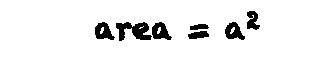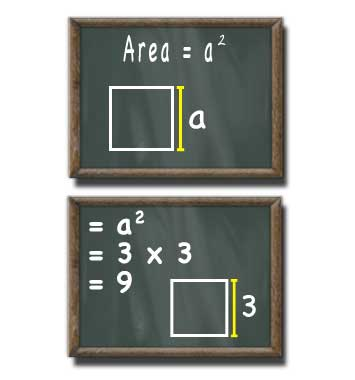# Square Area Calculator

## Square Area

#### For Squares with a Known Side

Result:
9Side (a) units
decimals

A square with a side length of 3 units has an area of 9 square units.

This calculator and more easy to use calculators waiting at www.KylesCalculators.com### Calculating the Area of a Square:

The area of a square with a known side length can calculated using the following formula:

A = a^2

The symbol ^2 means to square the item preceding it, in this case the side length (a). To square the side length multiply it by itself: a * a.

Below is an example of how to find the area of a square with the side length of 3.

• A = a^2
• = 3 * 3
• = 9